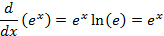Derivative of an Exponential

An important result in Calculus is the rate of change of the exponential function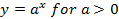As a first step we define a change in “y” for a small change in “x” as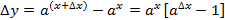And the slope isConsidering the definition of a logarithm, we can write in general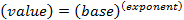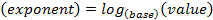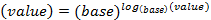or specifically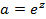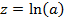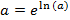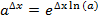Then we have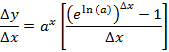Using a result for the derivation of the derivative of the logarithm we can express “e” as a limit as follows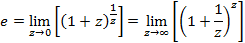and if we set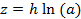then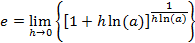Combining everything, we can apply the differential operator as follows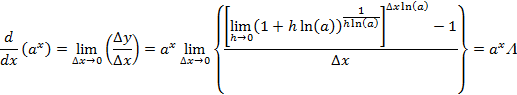Where we can simplify the new variable further, as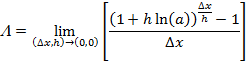And we can use a particular path for achieving the limit of (Δx, h) -> (0,0) by setting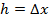, asso that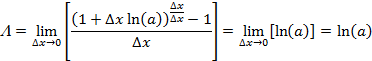Note that this result would be the same if we used any other arbitrary path as for example,, asso that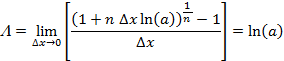Although this is more difficult to verify, one could put this on a spreadsheet to give confidence in the result even if this was not mathematically rigorous.

In any event the final result isor for the special case when the base is the constant “e”